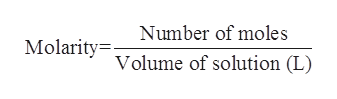How many moles of chloride ions are present in a 460.0 mL solution of CaCl2 that has a concentration of 0.270 M?

Question

How many moles of chloride ions are present in a 460.0 mL solution of CaCl2 that has a concentration of 0.270 M?

Step 1

The number of moles for a given chemical species refers to the ratio of mass to the molar mass. The mathematical formula which is used to calculate the number of moles is as follows:

Step 2

The molarity of a solution is defined as the ratio of the number of moles of solute present per liter of the solution. Its SI units are moles/liter.help_outlineImage TranscriptioncloseNumber of moles Molarityolume of solution (L) fullscreen
Step 3

The molarity of the calcium chloride solution is given to be 0.270 M.

The volume of the solution is give...

Want to see the full answer?

See Solution

Want to see this answer and more?

Our solutions are written by experts, many with advanced degrees, and available 24/7

See Solution
Tagged in

Inorganic Chemistry